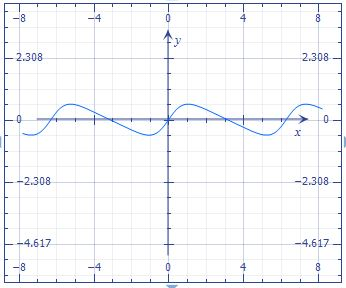# Draw the following function and find its critical points (if any). f(x) = \frac {\sin...

## Question:

Draw the following function and find its critical points (if any).

{eq}f(x) = \frac {\sin x}{2-\cos x} {/eq}

## Critical Points:

We can find the critical points of a function when we equal the first derivative to zero or when the first derivative does not exist. Also, we can classify the critical points found in maximum and minimum.

We have the function

{eq}f(x) = \frac {\sin x}{2-\cos x} {/eq}

Differentiating the function

{eq}f'(x)={\frac {\cos \left( x \right) }{2-\cos \left( x \right) }}-{\frac { \left( \sin \left( x \right) \right) ^{2}}{ \left( 2-\cos \left( x \right) \right) ^{2}}} {/eq}

{eq}f'(x)=0 {/eq} when {eq}x= \frac{5}{3} \pi \\ x= \frac{1}{3} \pi \\ x=- \frac{1}{3} \pi \\ x= - \frac{5}{3} \pi \\ x=\frac{7}{3} \pi \\ x=-\frac{7}{3} \pi \\ {/eq}

Critical points at:

{eq}( \frac{5}{3} \pi , - \frac{1}{3} \sqrt{ 3} ) \\ (- \frac{5}{3} \pi , \frac{1}{3} \sqrt{ 3} ) \\ (\frac{1}{3} \pi, \frac{1}{3} \sqrt{ 3}) \\ ( -\frac{1}{3} \pi, - \frac{1}{3} \sqrt{ 3}) \\ (\frac{7}{3} \pi , \frac{1}{3} \sqrt{ 3} ) \\ (-\frac{7}{3} \pi , - \frac{1}{3} \sqrt{ 3} ) \\ {/eq}

Draw the function: